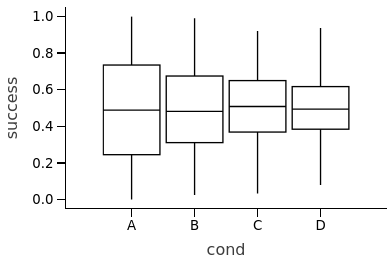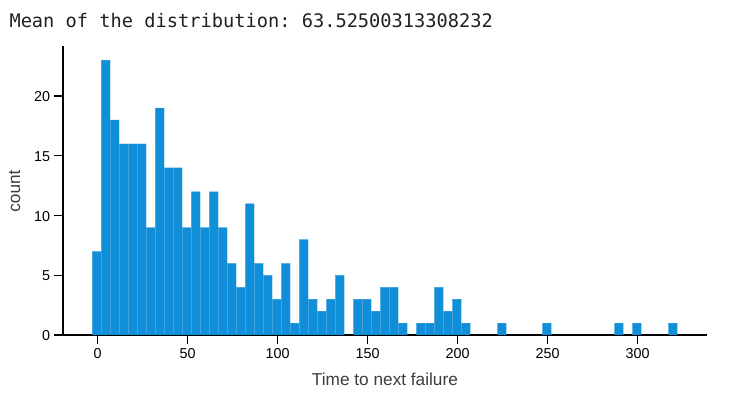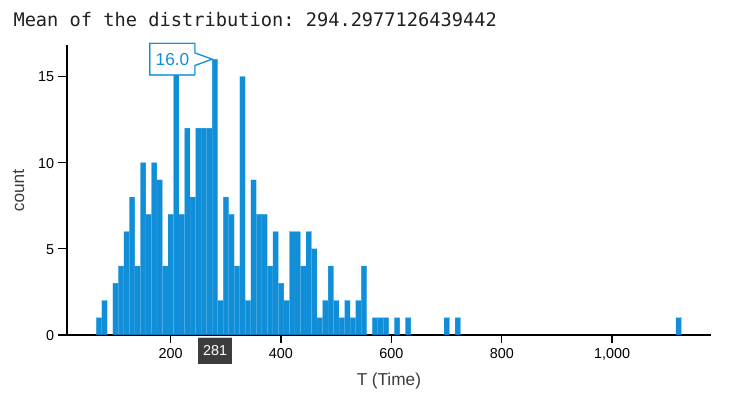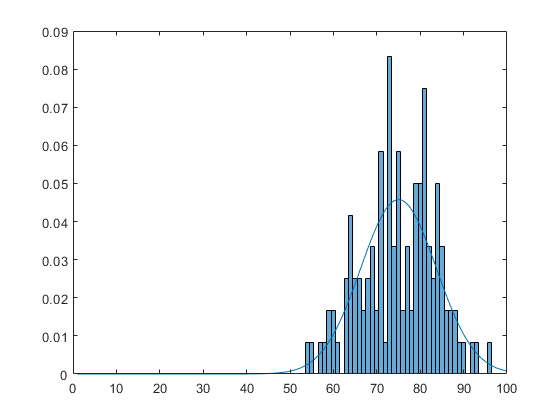So far, we have learnt that ITS and ARS are generic methods that extract random numbers from all probability distributions. However, it is inefficient due to it indirectness and calculations required.

Here are some faster methods to generate RNGs directly from “popular” known distributions.

NmDev’s S2 can generate RNGs from many distributions: Gaussian/normal, beta, gamma, exponential, poisson, binomial, rayleigh etc.

## Distributions to describe yes/no (boolean) events

### (Beta and Binomial Distributions)

Binomial DistributionEver been faced with a yes/no question? Binomial Distributions are used to find out the number of “yes”-es when these question is asked on many “people”/scenarios!

Unlike other distributions such as the exponential distribution, you need to know the total number of times you conduct the yes/no trials.

A common way to show this is the probability mass function!$\Pr(X = k) = \binom{n}{k}p^k(1-p)^{n-k}$

where:

• n is the number of trials (occurrences)
• k is the number of successful trials
• p is probability of success in a single trial
• Pr(X = k) Probability Mass function

To understand this nasty looking equation, let us imagine a scenario:

It is your school’s 50th anniversary and it needs a new face! 🏫 You propose a new school badge. You have to conduct the survey on foot as you know that no one reads their emails!

To get a accurate depiction of everyone’s opinion, it is almost impossible to survey everyone in the school. Hence, you want to model your school based on a sample of 100 students. This is possible because you are certain that the opinion of each student is independent of the previous one! Each student either 👍 or 👎 the logo.

1. 💬 “I think this school logo is not good. It’s too messy in design”
2. 💬 ” This school logo has a refreshing design! I like it!”
3. 💬 “I think this school logo looks pretty bad! The colours don’t contrast well. I prefer our current one more”.

You are dispirited that 2/3 students so far did not like the badge. You wonder if whether meeting students whom dislike the badge first will affect your sample results!

Thus, you realise that how you meet the students does not matter as they have been accounted for in the calculations (for binomial distribution). Hence, you continue your survey, in hope that more will approve your design.

⏰ 1 month later…

You now have the results, 62 out of 100 students are agreeable to the proposal. This translates to a 0.62 probability of success!

At the end of your survey, your teacher remarks that she would be willing to accept the new logo design if there is a 10% probability that less than 3000 (number of successful trials) of at least 5000 (total number of trials) of students in your school are likely to be agreeable. You plug the numbers into the formula above and ….

Wait! You can’t use this formula! This is a PMF, meant only for a specific value. Ah, so this is why we need to decide on the appropriate functions with the specific distribution that we choose to use. In this case, we will use other methods such as the Z-Test (which we will not go into detail on). We also cannot use the quantile function as it does not take into account confidence intervals of a population deduced from a sample, and hence Z-Test would be preferred.

Nevertheless, let’s explore what a quantile function does! Given a certain probability, we can work out the percentile whereby people are agreeable to the new proposal “success”

Note: The quantile function in NMDev actually divides a probability distribution into infinite continuous intervals with equal probabilities based on the inverse cumulative distribution function. This is not to be confused with quartile, which splits the distribution into four equal sections.

				
%use s2
var dist = BinomialDistribution(100,0.62)
// 90th percentile where 90% of data have values less than this variable
// in other words, 10th percentile is where 90% of data have values more than this variable
dist.quantile(0.1)


				
56.0
// 90% probability that 56 of 100 people agreeable to this proposal in this sample



#### Beta Distribution$\alpha$ parameter – refers to the number of successes$\beta$ parameter – refers to the number of failures

The probability density function for beta distribution is given below$\frac{1}{\beta(\alpha,\beta)}\cdot{x^{\alpha-1}(1-x)^{\beta-1}}$

where$\frac{1}{\beta(\alpha,\beta)} = \frac{\Gamma(\alpha+\beta)}{\Gamma(\alpha)\Gamma(\beta)}$$\Gamma$ represents the gamma function

Notice that the parameters${x^{\alpha-1}(1-x)^{\beta-1}}$ looks suspiciously similar to that of the binomial distribution$\binom{n}{k}p^k(1-p)^{n-k}$. While binomial distributions aims to model the number of successes given a certain probability$p$, the beta distribution models probability of random variable being successful.Here in the beta distribution, the independent variable (or probability in binomial distribution) is the random variable

The following image reflects the changes as the number of successes$\alpha$ or failures$\beta$ are changedWe can further see how this translates our understanding of the distribution by showing it through a box plot. A box plot is useful to showing the spread and mean as the values change.

(α,β)
👈 A: (1.0, 1.0)
👈 B: (2.0, 2.0)
👈 C: (3.0, 3.0)
👈 D: (4.0, 4.0)

Our spread in this box plot *slowly* reduces as the α,β values increases. Why is that? Meanwhile, the mean stays relatively the same

Next, we look at another example of a decreasing beta.👈 A: (1.0, 1.0)
👈 B: (1.0, 2.0)
👈 C: (1.0, 3.0)
👈 D: (1.0, 4.0)

Observe how the mean of of the box plot gradually decreases, indicating a decrease in “success”.

Now, hold for a moment. What would the box plot look like if alpha is increased while beta is kept constant? Remember that alpha measures the success of the distribution.

Try it out in kotlin (the code is below)! 😉 You can hover over the graph to get the specific stats.

Code for Creation of Box-plot as shown above

Code is run one after another – in the jupyter cells

Side Note: We will be using the double format, a 64-bit storage type, as they are a closest for storing an approximation of a real number, which helps in improving statistical accuracy.

Code to create a beta distribution in NmDev S2

We chose the Cheng 1978 algorithm (there is another one for beta distribution sampling called VanDerWaerden1969) as it is generally faster. [Refer to the book for more information]

				
// change the alpha and beta values accordingly
val alpha:Double = 0.1
val beta:Double = 0.2
var rng = Cheng1978(alpha, beta, new UniformRNG())




We can also generate a sequence of random numbers by sampling from the beta distribution, and compare the mean, variance, skew and kurtosis with the “theoretical” distribution.

				
theoretical mean = 0.333333, sample mean: 0.333094; N: 10000000
theoretical variance = 0.170940, sample var: 0.170869, stdev: 0.413363, N: 10000000
theoretical skew = 0.701066, sample skew: 0.702149; N: 10000000
theoretical kurtosis = -1.304348, sample kurtosis: -1.302781; N: 10000000



Why use a beta distribution? The parameters of number of successes or failures can easily be adjusted.

This is useful if you only have a limited test run, and are only able to get a certain number of data. As time goes on, the successes or failures can be updated, whilst still allowing random numbers generated to reflect the changes.

## Distributions relating to the Poisson Process

What is a poisson process? It basically helps us to describe events which occur independently in the future with a given frequency or rate set by us!Exponential Distribution

Trains in Singapore travelled about 26.7 million km in year 2020, about 73150km per day.

For simplicity sake, we take the mean  distance traveled between breakdowns as their average of the train lines 4.322 million km between failure. This translates to a rate of 59 days between a failure longer than 5 minutes.

Note: This is not a good approximation as there are flaws. One of them is assuming a constant train ridership –> which affects the amount of distance covered!!

A Poisson distribution like the one we did earlier are good for monitoring number of events in a given time period (eg. day, year). If the number of events per unit time follows a Poisson distribution, then the amount of time between events follows the exponential distribution. Exponential Distributions are thus good for predicting success, failure or arrival times.

The probability density function for the exponential distribution is described like this:$f(x;\lambda) = \begin{cases} \lambda e^{-\lambda x} & x \ge 0, \\ 0 & x < 0. \end{cases}$

•$\lambda \ge 0$ is the rate parameter
• Exponential‘s parameter λ is the same as that of Poisson process (λ).

Using the example above, we can plot the exponential distribution describing the scenario of train breakdowns, where the failure rate is$\frac{1}{59}=0.0169$Note: I specify the number of bins in the histogram, even though it is not entirely necessary. You can play around with this value to see how the shape of the histogram changes

The mean stays fairly close to the occurence of failure that we gave earlier. Yet the distribution is fairly skewed towards the left.

Assumption: One assumption made in this distribution is that the “failure rate” does not take into account previous events. That is, if the train had broken down recently, the distribution will not be able to take that into account. You cant “backdate” as occurrence of events in this distribution are “independent”.

#### Gamma Distribution

Previously, we tried to “predict” or model the disruptions taking more than 5 minutes of trains on the MRT system. Let’s say that after the 5th disruption within a year, we will need to hire a expert from overseas to investigate the causes of the disruptions. Hence, we will want to find out when the 5th disruption will occur.

One can think of this distribution as a continuation of the previous one. Similar to the exponential distrbution, it takes into account a parameter λ which is the rate of events following the Poisson process. However, a new parameter called k is the number of events we are waiting for.

Let’s plot the distribution for this gamma distribution. Notice that the value of λ (in the code below) is 59.0 instead of 0.0169. The reason for this is that the event rate parameter of the gamma distribution can be characterised by either θ (the mean wait time) or λ (the frequency of the events in a given time period).

Thus to make it more intuitive to understand, we take the reciprocal of the event rate, which gives us the time till the occurrence of the 5th event!This allows us to visualise how likely we are to exceed 5x breakdowns within a given time period.

#### Normal (aka Gaussian) Distribution

All the previous distributions we learn have histograms which are either skewed left or right.

But in real-life…

Most independent random things that happen (eg. results of the most recent Science Test!) will tend towards a mean$\mu$, after a certain number of events have happened. This is possible even if the random event (eg. how hard I studied for a test) itself does not happen in a normal distributionThe spread or “width” of the bell curve is defined by its standard deviation .

The variance of a distribution is represented as$\sigma^2$.

For the standard normal distribution with mean of 0 and standard deviation of 1, almost 68% of the data falls within one standard deviation from mean and 95 % within 2 std. deviations.

In Kotlin, we can generate standard normal samples and normal samples based on a specified mean and variance$\sigma^2$, as shown below

				
%use s2

val uniform = MersenneTwister()
uniform.seed(1234567890L)

val rng1 = Zignor2005(uniform)
val N:Int = 1000

/* Create an array of size N which will
soon be used to store doubles*/
var arr1 = DoubleArray(N)

// kotlin range(s) are inclusive of both starting and ending boundaries
for (i in 0..(N-1)) {
arr1[i] = rng1.nextDouble()
}

//check the statistics of the random samples
val var1 = Variance(arr1)

println("mean = %f, stdev = %f".format(var1.mean(), var1.standardDeviation()))

/* Repeat for the normal distribution */
val rng2 = NormalRNG(1.0,2.0,rng1) // mean = 1, std deviation = 2

/* Create an array of size N which will
soon be used to store doubles*/
var arr2 = DoubleArray(N)

// kotlin range(s) are inclusive of both starting and ending boundaries
for (i in 0..(N-1)) {
arr2[i] = rng2.nextDouble()
}

//check the statistics of the random samples
val var2 = Variance(arr2)

println("mean = %f, stdev = %f".format(var2.mean(), var2.standardDeviation()))



				
mean = 0.011830, stdev = 1.009379
mean = 0.940225, stdev = 2.037228



Thinking time 🤔: Notice that the values are close to their respective means and variance. What happens if you increase$N$, the number of samples?